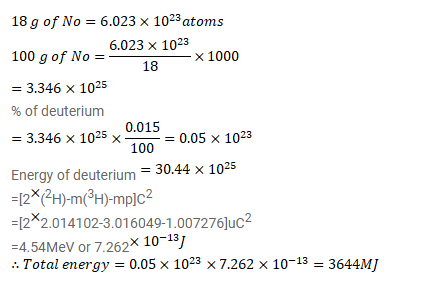# Calculate the energy that can be obtained from 1 kg of water through the fusion reaction

Question:

Calculate the energy that can be obtained from $1 \mathrm{~kg}$ of water through the fusion reaction ${ }^{2} \mathrm{H}+{ }^{2} \mathrm{H}^{3} \mathrm{H}+\mathrm{p}$

Assume that $1.5 \times 10^{-2} \%$ of natural water is heavy water $\mathrm{D}_{2} \mathrm{O}$ (by number of molecules) and all the deuterium is used for fusion.

Solution: